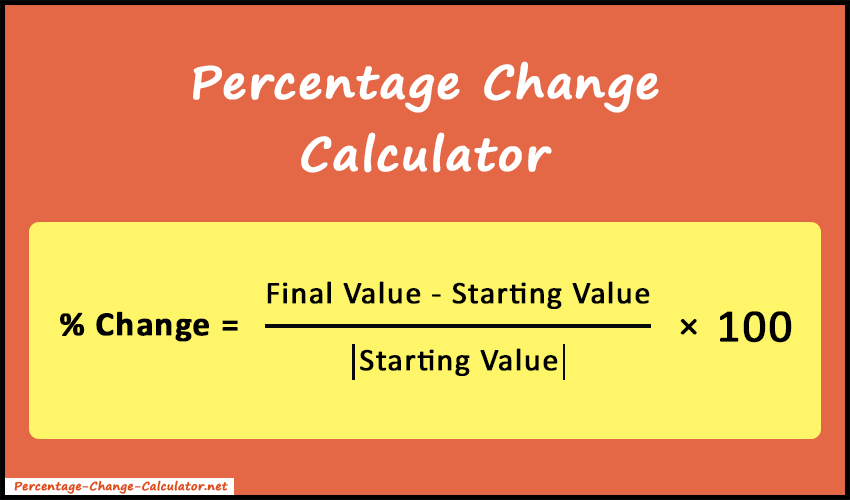# Percentage Change Calculator

The percentage change calculator is designed to calculate the percent(%) change (increase or decrease) between two given numbers.

%

The percentage change calculator is a free tool where you can determine the percent(%) change (increase or decrease) between the starting and final value. Also, it gives the difference between two given values and calculation steps.### What is Percentage Change?

It's a percent(%) increase or decrease measured between two values. For example, if the final value increases X% from the starting value, we can say there is an X% change. Similarly, if the final value decreases Y% from the starting value, we can say there is a -Y% change. The positive change denotes an increase and the negative change denotes a decrease.

### Percentage Change Formula

The percentage change is calculated using the following formula.

Percent Change =
 X2 - X1 |X1|
×  100

Where,
X1 = Starting value
X2 = Final value

Let's take some examples.

##### Example 1:

Suppose, the starting value is \$9500 and the final value is \$12000. Calculate the percentage change between given values.

##### Solution:

Here,
Starting value (X1) = \$9500,
Final value (X2) = \$12000.

Now, place the above values in the percentage change formula.

Percent Change =
 X2 - X1 |X1|
×  100
=
 12000 - 9500 9500
×  100   =  26.31%

So, there is a 26.31% increment between the starting and final values.

##### Example 2:

Suppose, a product cost is \$45 and there is a flat discount of \$10 on the selling price. Calculate how much percent you saved.

##### Solution:

Here,
Starting value (X1) = \$45,
Final value (X2) = \$45 - \$10 (flat discount) = \$35.

Percent Change =
 X2 - X1 |X1|
×  100
=
 35 - 45 45
×  100   =  -22.22%

Therefore, you saved 22.22% from the original price.

##### Example 3:

Suppose, we have a Starting value \$100 and a Percentage change 20%. Calculate the final value based on the given parameters.

##### Solution:

Here,
Starting value (X1) = \$100,
Percent Change = 20%.

Percent Change =
 X2 - X1 |X1|
×  100
20% =
 X2 - 100 |100|
×  100

X2 = 20 + 100 = 120

So, after a 20% change, the final value will be \$120.

Also, you can use the following formula to directly calculate the final value.

Final Value(X2) =
 6X1 5
=
 6 × 100 5

= 120

Our percentage change calculator can make your math calculations easier, faster, and more accurate. So, keep it handy and bookmark it for regular use.